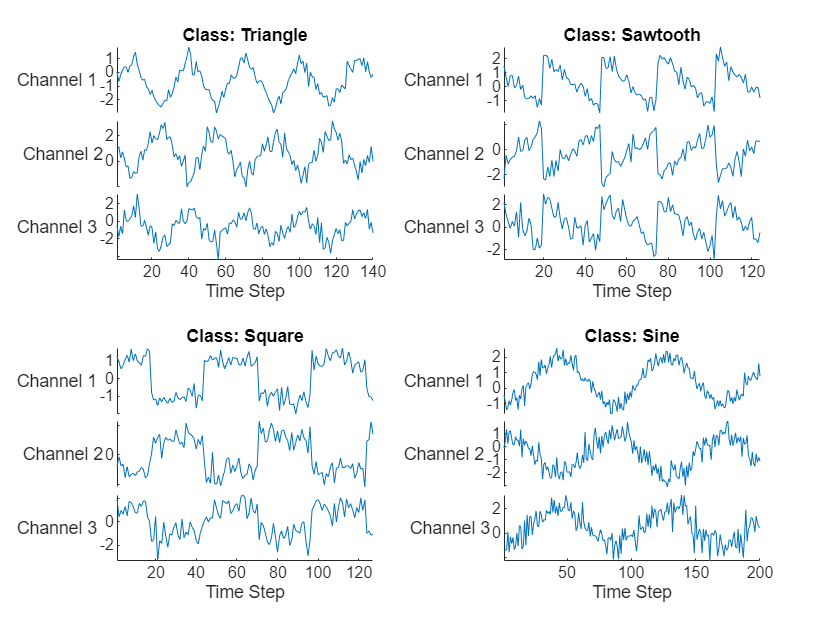# Sequence Classification Using 1-D Convolutions

This example shows how to classify sequence data using a 1-D convolutional neural network.

To train a deep neural network to classify sequence data, you can use a 1-D convolutional neural network. A 1-D convolutional layer learns features by applying sliding convolutional filters to 1-D input. Using 1-D convolutional layers can be faster than using recurrent layers because convolutional layers can process the input with a single operation. By contrast, recurrent layers must iterate over the time steps of the input. However, depending on the network architecture and filter sizes, 1-D convolutional layers might not perform as well as recurrent layers, which can learn long-term dependencies between time steps.

This example uses the Japanese Vowels data set described in  and . This example trains a 1-D convolutional neural network to recognize the speaker given time series data representing two Japanese vowels spoken in succession. The training data contains time series data for nine speakers. Each sequence has 12 features and varies in length. The data set contains 270 training observations and 370 test observations.

Load the example data from `WaveformData.mat`. The data is a `numObservations`-by-1 cell array of sequences, where `numObservations` is the number of sequences. Each sequence is a `numChannels`-by`-numTimeSteps` numeric array, where `numChannels` is the number of channels of the sequence and `numTimeSteps` is the number of time steps of the sequence and is labeled with the type of waveform.

`load WaveformData`

Visualize some of the sequences in a plot.

```numChannels = size(data{1},1); idx = [3 4 5 12]; figure tiledlayout(2,2) for i = 1:4 nexttile stackedplot(data{idx(i)}',DisplayLabels="Channel "+string(1:numChannels)) xlabel("Time Step") title("Class: " + string(labels(idx(i)))) end```Set aside data for validation and testing. Partition the data into a training set containing 80% of the data, a validation set containing 10% of the data, and a test set containing the remaining 10% of the data. To partition the data, use the `trainingPartitions` function, attached to this example as a supporting file. To access this file, open the example as a live script.

```numObservations = numel(data); [idxTrain,idxValidation,idxTest] = trainingPartitions(numObservations, [0.8 0.1 0.1]); XTrain = data(idxTrain); TTrain = labels(idxTrain); XValidation = data(idxValidation); TValidation = labels(idxValidation); XTest = data(idxTest); TTest = labels(idxTest);```

### Define 1-D Convolutional Network Architecture

Define the 1-D convolutional neural network architecture.

• Specify the input size as the number of features of the input data.

• Specify two blocks of 1-D convolution, ReLU, and layer normalization layers, where the convolutional layer has a filter size of 5. Specify 32 and 64 filters for the first and second convolutional layers, respectively. For both convolutional layers, left-pad the inputs such that the outputs have the same length (causal padding).

• To reduce the output of the convolutional layers to a single vector, use a 1-D global average pooling layer.

• To map the output to a vector of probabilities, specify a fully connected layer with an output size matching the number of classes, followed by a softmax layer and a classification layer.

```filterSize = 5; numFilters = 32; numFeatures = size(XTrain{1},1); numClasses = numel(categories(TTrain)); layers = [ ... sequenceInputLayer(numFeatures) convolution1dLayer(filterSize,numFilters,Padding="causal") reluLayer layerNormalizationLayer convolution1dLayer(filterSize,2*numFilters,Padding="causal") reluLayer layerNormalizationLayer globalAveragePooling1dLayer fullyConnectedLayer(numClasses) softmaxLayer classificationLayer];```

### Specify Training Options

Specify the training options:

• Train for 60 epochs using the Adam optimizer with a learn rate of 0.01.

• Validate the network using the validation data.

• Monitor the training progress in a plot and suppress the verbose output.

```miniBatchSize = 27; options = trainingOptions("adam", ... MaxEpochs=60, ... InitialLearnRate=0.01, ... SequencePaddingDirection="left", ... ValidationData={XValidation,TValidation}, ... Plots="training-progress", ... Verbose=0);```

### Train Network

Train the network with the specified training options using the `trainNetwork` function.

`net = trainNetwork(XTrain,TTrain,layers,options);`### Test Network

Classify the test data using the same sequence padding options used for training.

```YTest = classify(net,XTest, ... SequencePaddingDirection="left");```

Calculate the classification accuracy of the predictions.

`acc = mean(YTest == TTest)`
```acc = 0.8400 ```

Visualize the predictions in a confusion matrix.

```figure confusionchart(TTest,YTest)```